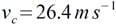Despite being in the twilight of an illustrious career, few footballers are more recognisable that David Beckham. Whether playing for Manchester United, Real Madrid, AC Milan, LA Galaxy or England, his ability to score from a free-kick is legendary. With many predicting that Beckham’s swansong will be captaining the 2012 British Olympic Football Team, here is a look at the mathematics behind the efforts of all those who have tried to “bend it like Beckham”.

Let’s imagine a typical Beckham-esque free kick from the just outside the ‘D’ on the edge of the penalty area around 30 metres from goal. Beckham knows that if he puts side spin on the ball, so that it rotates anti-clockwise, then he can get the ball to curve from right to left, avoiding the wall and perplexing the goalkeeper. Looking down from above we can see why:In this scenario the air travels faster on the left hand side of the ball because it is travelling in the same direction as the ball’s spin – it is dragged along by the ball. On the right hand side, the ball is spinning against the direction of air flow and so the air travels slower over that part of the ball.

In fluid dynamics, Bernoulli’s Principle states that if the velocity of a fluid is increased then a drop in pressure results. The opposite is also true. This means that, in the above scenario, air pressure drops on the left hand side of the ball and increases on the right. The pressure difference creates a force acting away from the area of high pressure – the ball moves to the left.

This force is called the Magnus force after German physicist Heinrich Magnus who was originally trying to explain why spinning shells and bullets deviate from their original trajectories.

There is another factor, however, that can be used to deceive the goalkeeper. In fluid dynamics there is a cut off point where the flow of the fluid – in this case the air over the ball – changes from a smooth flow called “laminar flow” to “turbulent”. Turbulent flows create eddies and other instabilities in the flow of air which can cause the ball to suddenly deviate from the trajectory that it was travelling.

The change from laminar to turbulent flow happens at a critical value of the ball’s velocity. The value of this critical velocity is dependent upon a dimensionless number called the Reynold’s Number. In the case of a sphere, the critical velocity, vc, and the Reynold’s Number, Rc, are related by the following equation:where ? is the viscosity of air, ? is the density of air and r is the radius of the ball.

We can use some typical values to calculate the velocity at which the flow over the football becomes turbulent and the ball’s behaviour is more likely to worry the goalkeeper.

? = 1.78 x 10-5 kg m-1 s-1 (at 15°C)

? = 1.225 kg m-3 (at 15°C, at sea level)

r = 0.11m (for a standard Size 5 ball used by professional players)

Rc ~ 4 x 105 (dimensionless)

Plugging these numbers into equation (1) givesThis is around 60 miles per hour.

Considering 30 metre free kicks reach the goal around one second after the ball was struck, it is clear that professional footballers can strike a ball at this velocity. At such a pace the flow becomes turbulent and the ball is more likely to move in flight, on top of the swerve resulting from the Magnus Force.

But just how much can the ball swerve in flight?

The lift force, FL, on the ball is given by:where Acs is the cross-sectional area of the ball and CL is the dimensionless “lift coefficient”

Re-written in terms of the radius of the ball the equation reads:

A typical value for CL in this case is 0.05. Using that, and the values above, gives:

Using Newton’s Second Law of Motion, along with the mass of a typical football, mb, allows calculation of the ball’s acceleration, ?:Official Size 5 footballs must have a mass between 410 and 450 grams. Plugging the mid-point, 430 grammes, into equation (5):

Finally, using an equation of kinematics, we can work out smax : the maximum amount the ball could displace from its course during its one second flight from boot to back of the net:where u is the ball’s initial velocity and t is time.

As the ball is initially at rest, the equation simplifies to:So not only does a goalkeeper have to face the uncertainty of a ball that curls around the wall thanks to the Magnus Force, he also has to think about deviations of up to four metres due to the turbulent flow of air over a ball travelling faster than the critical velocity.

If David Beckham’s legendary right boot scores a free kick that wins the Gold Medal for the British team at 2012 Olympics, spare a thought for the maths behind the victory.